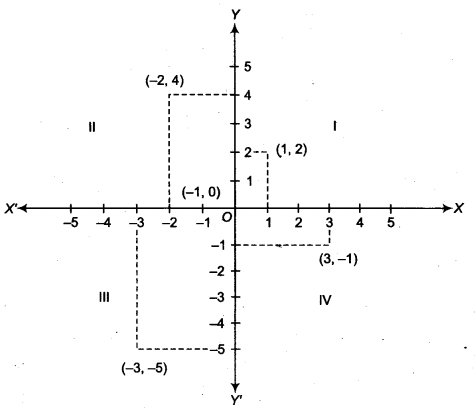NCERT Solutions for Class 9 Maths Chapter 6 Coordinate Geometry Ex 6.3 are part of NCERT Solutions for Class 9 Maths. Here we have given NCERT Solutions for Class 9 Maths Chapter 6 Coordinate Geometry Ex 6.3.

 Board CBSE Textbook NCERT Class Class 9 Subject Maths Chapter Chapter 6 Chapter Name Coordinate Geometry Exercise Ex 6.3 Number of Questions Solved 2 Category NCERT Solutions

## NCERT Solutions for Class 9 Maths Chapter 6 Coordinate Geometry Ex 6.3

Question 1.
In which quadrant or on which axis does each of the points (-2,4), (3, – 1), (-1,0), (1, 2) and (-3, – 5) lie? Verify your answer by locating them on the cartesian plane.
Solution:(i) (-2, 4) lies in II quadrant.
(ii) (3, -1) lies in IV quadrant.
(iii) (-1,0) lies on X-axis.
(iv) (1, 2) lies in I quadrant.
(v) (-3, -5) lies in III quadrant.

Question 2.
Plot the points (x, y) given in the following table on the plane, choosing suitable units of distance on the axes.Solution:
Let 1 unit = 1 cm, then positions of given points in the cartesian plane are given below.We hope the NCERT Solutions for Class 9 Maths Chapter 6 Coordinate Geometry Ex 6.3 help you. If you have any query regarding NCERT Solutions for Class 9 Maths Chapter 6 Coordinate Geometry Ex 6.3, drop a comment below and we will get back to you at the earliest.